## Abstract

The present article investigates the squeezing flow of two types of nanofluids such as Cu-water and Cu-kerosene between two parallel plates in the presence of magnetic field. The governing non-linear partial differential equations are transformed into ordinary differential equations by applying suitable similarity transformation and then solved numerically using RK-4 method with shooting technique and analytically using differential transformation method (DTM). The influence of arising relevant parameters on flow characteristics has been discussed through graphs and tables. A comparative study has been taken into account between existing results and present work and it is found to be in excellent harmony.

## Keywords

Squeezing flow; Nanofluid; Magnetic field; Differential transformation method

## 1. Introduction

The squeezing flow of Newtonian and non-Newtonian fluids continues to stimulate significant interest by the researchers owing to increasing application in different fields of engineering, technology such as polymer processing, transient loading of mechanical components, compression, the squeezed film in power transmission, moving pistons, and chocolate filler. Such flows are performed between two moving parallel plates. Classical work in this sector was first reported by Stefan  considering the squeezing flow using lubrication approach in 1874. After that Reynolds  considered the problems for elliptic plates in 1886. Archibald  analyzed the squeezing flow through rectangular plates in 1956. Gradually day by day considerable efforts have been devoted by the researchers to avail the squeezing flow easier and reliable. Rashidi et al.  discussed the two dimensional axisymmetric squeezing flow between parallel plates. Effects of magnetic field in the squeezing flow between infinite parallel plates were reported by Siddiqui et al. . Hamdan and Baron  investigated the squeezing flow of dusty fluid between parallel disks and discussed the squeezing effects on the velocity profiles. Domairry and Aziz  delivered the approximate analytic solution for the squeezing flow of viscous fluid between parallel disks with suction or blowing. Hayat et al.  extended the work of Domairry and Aziz  to analyze the squeezing flow of non-Newtonian fluids by taking second grade fluids.

Recently the study of heat transfer characteristics of squeezing flow of viscous fluid has gained considerable attention owing to its various applications in many branches of science and engineering. The squeezing flow through a porous surface has been addressed by Mahmood et al. . Investigation reveals that magnitude of local Nusselt number increases with Prandtl number. The results are satisfied with Mustafa et al. . Heat transfer characteristics in a squeezing flow between parallel disks has been studied by Duwairi et al. . Khaled and Vafai  analyzed the hydromagnetic effects on flow and heat transfer over a horizontal surface placed in an externally squeezed free stream. They found that Nusselt number and wall shear stress are both increasing functions of the magnetic parameter. Analytical investigation of the unsteady squeezing flow of viscous Jeffery fluid between parallel disks was performed by Qayyum et al.  and discussed the porosity and squeezing effects on the velocity profiles.

The modern age is rightly called the age of science. Whenever we cast our eyes, we see that ongoing advanced technology requires further improvement of heat transfer from energy saving point of view. Since the conventional heat transfer fluids such as water, kerosene, and ethylene glycol have low thermal conductivity, modern science bestowed blessing in the form of nanofluid in which nano-sized particles are added to the base fluid to increase the heat transfer capabilities of the base fluid. It should be noticed that there have been published several recent papers , , ,  and  on the mathematical and numerical modeling of convective heat transfer mechanism in nanofluids. Mandy  investigated mixed convection flow and heat transfer of nanofluids due to an unsteady stretching sheet. These models have some advantage over experimental studies due to many factors that influence nanofluid properties. Recently, nanofluid flow and heat transfer characteristics under different flow configuration were discussed by Hatami et al.  and . Analytical investigation of unsteady squeezing nanofluid flow has been discussed by Pourmehran et al. . They showed that highest value of Nusselt number can be obtained by selecting silver as nanoparticle. All the abovementioned models are single phase model. Recently a new dimension has been added by  and  in terms of complex geometry or two phase model for simulating nanofluid.

Nowadays modern science and engineering fields are blessed with so many numerical and analytical methods to obtain accurate approximate solutions from non-linear equations. The methods are variation of parameter method (VPM)  and , differential transformation method (DTM) , , ,  and , adomian decomposition method (ADM)  and , homotopy analysis method (HAM)  and , homotopy perturbation method (HPM) , etc. In this article we have applied relatively a novel analytic technique, say differential transformation method (DTM) which can be said as a modified version of Taylor series method. Zhou  was the first to initiate the differential transformation method (DTM). It is the method in which we determine the coefficient of Taylor series of the function by solving the recursive equation from the given differential equation. While the Taylor series method requires very time consuming symbolic computation and derivatives of the data function for higher order equations, DTM plays a powerful authority to obtain the solution in a purely numerical way providing a smooth, functional form within a very few steps. Another essential advantage of this DTM method is that it reduces the size of computational work providing the desired accuracy together with fast convergence rate. More or less every semi analytical technique is helpful to obtain solutions but DTM is free from any linearization, perturbation, discretization or restrictive assumption like HAM, HPM, etc. That’s why it is not affected due to any round off errors. Hence it can be applied directly to linear and non-linear ordinary differential equations as a promising tool.

Motivated by the above investigations the present paper deals with the squeezing flow of Cu-water and Cu-kerosene nanofluid considering the presence of external applied magnetic field. Similarity transformation has been used to obtain ordinary differential equations from the governing equations. The reduced ordinary differential equations have been solved by DTM as well as by RK-4 method with shooting technique.

## 2. Mathematical formulation

### 2.1. Flow analysis

Consider the viscous incompressible nanofluid flow and heat transfer in a two dimensional co-ordinate system. The co-ordinate system is chosen in such a way that x-axis is measured along the plate and y axis is normal to the plate as shown in Fig. 1. The squeezing flow has been performed through a system having two parallel plates situated at ${\textstyle h(t)=H{\left(1-\alpha t\right)}^{1/2}}$ distance apart where ${\textstyle \alpha >0}$ refers to the squeezing movement of both plates with velocity ${\textstyle v(t)={\frac {dh}{dt}}}$ until they touch each other at ${\textstyle t={\frac {1}{\alpha }}}$. Also ${\textstyle \alpha <0}$ refers to movement of the plate away to each other and ${\textstyle \alpha }$ is called the characteristic parameter having dimension of time inverse. H is the initial position of the plate at time t = 0. A uniform magnetic field of strength ${\textstyle B(t)=B_{0}{\left(1-\alpha t\right)}^{-1/2}}$ is applied normal to the plate where ${\textstyle B_{0}}$ is the initial intensity of the magnetic field. In the mathematical formulation scheme we proceed with the 0 following assumption that there is no chemical reaction, radiative heat transfer, nanoparticles and base fluid are in thermal equilibrium and no slip occurs between them. All body forces are assumed to be neglected. Here we have considered two types of nanofluids cu-water and cu-kerosene. The thermophysical properties of the nanofluid are given in Table 1.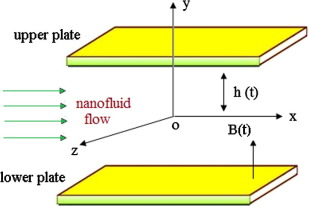Figure 1. Physical model of the problem.

Table 1. Thermophysical properties of water, kerosene, copper nanoparticles.
${\textstyle \rho }$ (kg/m3) ${\textstyle C_{p}}$ (J/kg K) ${\textstyle \kappa }$ (W/m K)
Pure water 997.1 4179 0.613
Kerosene 783 2090 0.145
Copper 8933 385 401

Under the above stated situation the governing equations are as follows:

 ${\frac {\partial u}{\partial x}}+{\frac {\partial v}{\partial y}}=$$0{\mbox{,}}$
(1)

 ${\rho }_{nf}\left({\frac {\partial u}{\partial t}}+u{\frac {\partial u}{\partial x}}+\right.$$\left.v{\frac {\partial u}{\partial y}}\right)=-{\frac {\partial p}{\partial x}}+$${\mu }_{nf}\left({\frac {{\partial }^{2}u}{\partial x^{2}}}+\right.$$\left.{\frac {{\partial }^{2}u}{\partial y^{2}}}\right)-$$\sigma B^{2}(t)u{\mbox{,}}$
(2)

 ${\rho }_{nf}\left({\frac {\partial v}{\partial t}}+u{\frac {\partial v}{\partial x}}+\right.$$\left.v{\frac {\partial v}{\partial y}}\right)=-{\frac {\partial p}{\partial y}}+$${\mu }_{nf}\left({\frac {{\partial }^{2}v}{\partial x^{2}}}+\right.$$\left.{\frac {{\partial }^{2}v}{\partial y^{2}}}\right){\mbox{,}}$
(3)
 ${\frac {\partial T}{\partial t}}+u{\frac {\partial T}{\partial x}}+$$v{\frac {\partial T}{\partial y}}={\frac {k_{nf}}{{\left(\rho C_{p}\right)}_{nf}}}\left({\frac {{\partial }^{2}T}{\partial x^{2}}}+\right.$$\left.{\frac {{\partial }^{2}T}{\partial y^{2}}}\right)+$${\frac {{\mu }_{nf}}{{\left(\rho C_{p}\right)}_{nf}}}\left[4{\left({\frac {\partial u}{\partial x}}\right)}^{2}+\right.$$\left.{\left({\frac {\partial u}{\partial y}}+{\frac {\partial v}{\partial x}}\right)}^{2}\right]{\mbox{,}}$
(4)

where u, v are the velocity components in x and y   directions respectively. Here ${\textstyle T{\mbox{,}}p{\mbox{,}}{\rho }_{nf}{\mbox{,}}{\mu }_{nf}{\mbox{,}}{\left(\rho C_{p}\right)}_{nf}{\mbox{,}}k_{nf}}$ represents the temperature, pressure, effective density, effective dynamic viscosity, effective heat capacity and effective thermal conductivity of the nanofluids respectively. Now the relations between base fluid and nanoparticles are given by

 ${\rho }_{nf}=(1-\phi ){\rho }_{f}+\phi {\rho }_{s}{\mbox{,}}$
(5)
 ${\left(\rho C_{p}\right)}_{nf}=(1-\phi ){\left(\rho C_{p}\right)}_{f}+$$\phi {\left(\rho C_{p}\right)}_{s}{\mbox{,}}$
(6)
 ${\mu }_{nf}={\frac {{\mu }_{f}}{{\left(1-\phi \right)}^{2.5}}}{\mbox{,}}$
(7)
 ${\frac {k_{nf}}{k_{f}}}={\frac {k_{s}+2k_{f}-2\phi (k_{f}-k_{s})}{k_{s}+2k_{f}+\phi (k_{f}-k_{s})}}{\mbox{,}}$
(8)

The relevant boundary conditions for the present problem are as follows:

 ${\begin{array}{l}u=0{\mbox{,}}v={\frac {dh}{dt}}{\mbox{,}}T=T_{H}\quad {\mbox{at}}\quad y=h(t)\\u=0{\mbox{,}}v=0{\mbox{,}}{\frac {\partial T}{\partial y}}=0\quad {\mbox{at}}\quad y=0\end{array}}$
(9)

Now in order to convert the partial differential equation into ordinary differential equations, let us introduce the dimensionless functions ${\textstyle f{\mbox{,}}\theta }$ and similarity variable ${\textstyle \eta }$ as follows:

 ${\begin{array}{l}u={\frac {\alpha x}{2(1-\alpha t)}}f^{'}(\eta ){\mbox{,}}\theta ={\frac {T}{T_{H}}}{\mbox{,}}v={\frac {-\alpha H}{\sqrt {1-\alpha t}}}f(\eta ){\mbox{,}}\\B(t)=B_{0}{\left(1-\alpha t\right)}^{-1/2}{\mbox{,}}\eta ={\frac {y}{H{\sqrt {1-\alpha t}}}}\end{array}}$
(10)

where prime denotes the differentiation with respect to ${\textstyle \eta }$. Using the above transformation and simplifying Eqs. (2) and (3) by eliminating pressure terms we have

 $f^{iv}-S\cdot A_{1}{\left(1-\phi \right)}^{2.5}(3f^{''}+$$\eta f^{'''}+f^{'}f^{''}-f\cdot f^{'''})-M^{2}f^{''}=$$0$
(11)

 ${\theta }^{''}+Pr\cdot S\cdot \left({\frac {A_{2}}{A_{3}}}\right)(f{\theta }^{'}-$$\eta {\theta }^{'})+{\frac {Pr\cdot Ec}{A_{3}{\left(1-\phi \right)}^{2.5}}}(f^{{''}2}+$$4{\delta }^{2}f^{{'}2})=0$
(12)

with the association of the boundary conditions

 ${\begin{array}{l}f^{''}(0)=0{\mbox{,}}f(0)=0{\mbox{,}}{\theta }^{'}(0)=0\quad {\mbox{for}}\quad \eta =0{\mbox{,}}\\f^{'}(1)=0{\mbox{,}}f(1)=1{\mbox{,}}\theta (1)=1\quad {\mbox{for}}\quad \eta =1\end{array}}$
(13)

where ${\textstyle A_{1}=(1-\phi )+\phi {\frac {{\rho }_{s}}{{\rho }_{f}}}{\mbox{,}}A_{2}=}$$(1-\phi )+\phi {\frac {{\left(\rho C_{p}\right)}_{s}}{{\left(\rho C_{p}\right)}_{f}}}{\mbox{,}}A_{3}=$${\frac {k_{s}+2k_{f}-2\phi (k_{f}-k_{s})}{k_{s}+2k_{f}+\phi (k_{f}-k_{s})}}{\mbox{,}}$${\textstyle S={\frac {\alpha H^{2}}{2{\nu }_{f}}}}$ denotes the squeeze number having the property S > 0 corresponds to the plates moving apart and S < 0 corresponds to the plates moving to each other, ${\textstyle Pr={\frac {{\mu }_{f}{\left(\rho C_{p}\right)}_{f}}{{\rho }_{f}k_{f}}}}$ is the Prandtl number, ${\textstyle Ec={\frac {{\rho }_{f}}{{\left(\rho C_{p}\right)}_{f}T_{H}}}{\left[{\frac {\alpha x}{2(1-\alpha t)}}\right]}^{2}}$ is the modified Eckert number, ${\textstyle M={\frac {{\sigma }_{nf}}{{\mu }_{nf}}}H^{2}\alpha B_{0}^{2}}$ is the Hartman number, and ${\textstyle \delta ={\frac {H(1-\alpha t)}{x}}}$ is the dimensionless length.

### 2.2. Physical quantities

For practical purpose the skin friction coefficient and Nusselt number can be defined respectively as follows:

 $C_{f}={\frac {{\mu }_{nf}{\left({\frac {\partial u}{\partial y}}\right)}_{y=h(t)}}{{\frac {1}{2}}{\rho }_{nf}{\left({\frac {dh}{dt}}\right)}^{2}}}{\mbox{,}}\quad Nu=$${\frac {-{Hk}_{nf}{\left({\frac {\partial T}{\partial y}}\right)}_{y=h(t)}}{k_{f}T_{H}}}$
(14)

In terms of dimensionless variables the reduced skin friction coefficient and the reduced Nusselt number are respectively given by

 ${\begin{array}{l}C_{fr}={\frac {{Re}_{x}C_{f}H^{2}{\sqrt {1-\alpha t}}}{2x^{2}}}={\frac {f^{''}(1)}{A_{1}{\left(1-\phi \right)}^{2.5}}}{\mbox{,}}\\{Nu}_{r}=Nu\cdot {\sqrt {1-\alpha t}}=-A_{3}{\theta }^{'}(1)\end{array}}$
(15)

where ${\textstyle {Re}_{x}={\frac {x\alpha H}{2{\nu }_{f}}}}$ is the local Reynolds number.

## 3. Numerical experiment

### 3.1. Differential transformation method (DTM)

We can define a function ${\textstyle g(\lambda )}$ in differential transformation method as follows:

 $G(k)={\frac {1}{k!}}{\left[{\frac {d^{k}g(\lambda )}{d{\lambda }^{k}}}\right]}_{\lambda ={\lambda }_{0}}$
(16)

where ${\textstyle G(k)}$ is called the T-function or simply transformed form of ${\textstyle g(\lambda )}$. The inverse transformation of ${\textstyle G(k)}$ is defined as

 $g(\lambda )=\sum _{k=0}^{\infty }G(k)\cdot {\left(\lambda -{\lambda }_{0}\right)}^{k}$
(17)

Now putting the value of ${\textstyle G(k)}$ in (17) as we defined earlier in (16) we can rewrite (17) as

 $g(\lambda )=\sum _{k=0}^{\infty }{\frac {1}{k!}}{\left[{\frac {d^{k}g(\lambda )}{d{\lambda }^{k}}}\right]}_{\lambda ={\lambda }_{0}}{\left(\lambda -{\lambda }_{0}\right)}^{k}$
(18)

In the upcoming solution procedure profile we have used some of the basic operations of DTM which we have listed in Table 2.

Table 2. Differential transformation of some functions.
Original function T-function
${\textstyle h(\lambda )={\frac {d^{m}g(\lambda )}{d{\lambda }^{m}}}}$ ${\textstyle H[k]={\frac {(k+m)!}{k!}}G[k+m]}$
${\textstyle h(\lambda )=g(\lambda ).p(\lambda )}$ ${\textstyle H[k]={\sum }_{n=0}^{k}G[n]P[k-n]}$
${\textstyle h(\lambda )={\lambda }^{n}}$ ${\textstyle H[k]=\delta (k-n)={\begin{array}{l}1{\mbox{,}}k=n\\0{\mbox{,}}k\not =n\end{array}}}$
${\textstyle h(\lambda )=\alpha g(\lambda )\pm \beta p(\lambda )}$ ${\textstyle H[k]=\alpha G[k]\pm \beta P[k]}$

### 3.2. Solution by DTM

Now applying DTM in Eq. (11), we can obtain

 ${\begin{array}{l}(k+1)(k+2)(k+3)(k+4)F[k+4]-{SA}_{1}{\left(1-\phi \right)}^{2.5}\sum _{m=0}^{k}(\Delta [k-m-1](m+1)(m+2)(m+3)F[m+3])\\-3{SA}_{1}{\left(1-\phi \right)}^{2.5}(k+1)(k+2)F[k+2]\\-{SA}_{1}{\left(1-\phi \right)}^{2.5}\sum _{m=0}^{k}((k-m+1)F[k-m+1](m+1)(m+2)F[m+2])\\+{SA}_{1}{\left(1-\phi \right)}^{2.5}\sum _{m=0}^{k}(F[k-m](m+1)(m+2)(m+3)F[m+3])-M^{2}(k+1)(k+2)F[k+2]=0\end{array}}$
(19)

where ${\textstyle \Delta (m)={\begin{array}{l}1{\mbox{,}}m=1\\0{\mbox{,}}m\quad \not =\quad 1\end{array}}}$

The corresponding boundary conditions are transformed as

 $F=0{\mbox{,}}F=k_{1}{\mbox{,}}F=0{\mbox{,}}F=k_{2}$
(20)

Similarly, we have from (12)

 ${\begin{array}{l}(k+1)(k+2)\Theta [k+2]+Pr\cdot S\cdot \left({\frac {A_{2}}{A_{3}}}\right)\sum _{m=0}^{k}(F[k-m](m+1)\Theta (m+1))\\-Pr\cdot S\cdot \left({\frac {A_{2}}{A_{3}}}\right)\sum _{m=0}^{k}(\Delta [k-m](m+1)\Theta (m+1))\\+{\frac {Pr\cdot Ec}{A_{3}{\left(1-\phi \right)}^{2.5}}}\sum _{m=0}^{k}((k-m+1)(k-m+2)F[k-m+2](m+1)(m+2)F[m+2])\\+{\frac {Pr\cdot Ec\cdot 4{\delta }^{2}}{A_{3}{\left(1-\phi \right)}^{2.5}}}\sum _{m=0}^{k}((k-m+1)F[k-m+1](m+1)F[m+1])\end{array}}$
(21)

where ${\textstyle \Delta (m)={\begin{array}{l}1{\mbox{,}}m=1\\0{\mbox{,}}m\quad \not =\quad 1\end{array}}}$

The relevant boundary conditions become

 $\Theta =k_{3}{\mbox{,}}\Theta =0$
(22)

where ${\textstyle F[k]{\mbox{,}}\Theta [k]}$ are the transformed form of ${\textstyle f(\eta ){\mbox{,}}\theta (\eta )}$ and ${\textstyle k_{1}{\mbox{,}}k_{2}{\mbox{,}}k_{3}}$ are the constants which can be obtained using the boundary condition. Now the iteration schemes are as follows:

 ${\begin{array}{l}F=0{\mbox{,}}F=k_{1}{\mbox{,}}F=0{\mbox{,}}F=k_{2}{\mbox{,}}F=0{\mbox{,}}\\F={\frac {3}{20}}S\cdot A_{1}{\left(1-\phi \right)}^{2.5}k_{2}+{\frac {1}{20}}M^{2}\cdot k_{2}{\mbox{,}}\\F={\frac {1}{360}}\left[\left(6+{\frac {9.M^{2}}{5}}\right)S\cdot A_{1}{\left(1-\phi \right)}^{2.5}k_{2}+{\frac {3}{5}}M^{4}\cdot k_{2}\right]{\mbox{,}}\ldots \end{array}}$
(23)

and

 ${\begin{array}{l}\Theta =k_{3}{\mbox{,}}\Theta =0{\mbox{,}}\Theta =-{\frac {Pr\cdot Ec\cdot 2{\delta }^{2}k_{1}^{2}}{A_{3}{\left(1-\phi \right)}^{2.5}}}{\mbox{,}}\Theta =0{\mbox{,}}\\\Theta =Pr\cdot S\cdot \left({\frac {A_{2}}{A_{3}}}\right)\left({\frac {1-k_{1}}{6}}\right)\cdot \Theta -{\frac {Pr\cdot Ec}{A_{3}{\left(1-\phi \right)}^{2.5}}}[4k_{2}^{2}+2k_{1}k_{2}{\delta }^{2}]{\mbox{,}}\ldots \end{array}}$
(24)

Substituting the above Eqs. (23) and (24) into the main equation based on DTM we can obtain the following solutions:

 $f(\eta )=k_{1}\eta +k_{2}{\eta }^{3}+\left({\frac {3}{20}}S\cdot A_{1}{\left(1-\phi \right)}^{2.5}k_{2}+\right.$$\left.{\frac {1}{20}}M^{2}\cdot k_{2}\right){\eta }^{5}+{\frac {1}{360}}\left[\left(6+\right.\right.$$\left.\left.{\frac {9\cdot M^{2}}{5}}\right)S\cdot A_{1}{\left(1-\phi \right)}^{2.5}k_{2}+\right.$$\left.{\frac {3}{5}}M^{4}\cdot k_{2}\right]{\eta }^{6}+\ldots$
(25)
 $\theta (\eta )=k_{3}-{\frac {Pr\cdot Ec\cdot 2{\delta }^{2}k_{1}^{2}}{A_{3}{\left(1-\phi \right)}^{2.5}}}{\eta }^{2}+$$\left[Pr\cdot S\cdot \left({\frac {A_{2}}{A_{3}}}\right)\left({\frac {1-k_{1}}{6}}\right)\cdot \Theta -\right.$$\left.{\frac {Pr\cdot Ec}{A_{3}{\left(1-\phi \right)}^{2.5}}}[4k_{2}^{2}+\right.$$\left.2k_{1}k_{2}{\delta }^{2}]\right]{\eta }^{4}+\ldots$
(26)

Considering ${\textstyle \eta =1{\mbox{,}}S=0.5{\mbox{,}}Pr=0.71{\mbox{,}}Ec=0.5{\mbox{,}}M=}$$0.5{\mbox{,}}\delta =0.1$ one can easily get ${\textstyle k_{1}=1.460028967{\mbox{,}}k_{2}=-0.420057934{\mbox{,}}k_{3}=}$$1.016183698{\mbox{.}}$

### 3.3. Solution by RK-4 method

Now we proceed to solve numerically the Eqs. (11) and (12) together with the boundary conditions (13) by switching them to an initial value problem. The Eqs. (11) and (12) can be written in the form of first order differential equations as follows:

 ${\begin{array}{l}f^{'}=z\\z^{'}=p\\p^{'}=q\\q^{'}=S\cdot A_{1}\cdot {\left(1-\phi \right)}^{2.5}(3p+\eta q+zp-fq)+M^{2}p\end{array}}$
(27)

and

 ${\begin{array}{l}{\theta }^{'}=r\\r^{'}=-Pr\cdot S\cdot {\frac {A_{2}}{A_{3}}}\cdot (fr-\eta r)-{\frac {Pr\cdot Ec}{A_{3}\cdot {\left(1-\phi \right)}^{2.5}}}\cdot (p^{2}+4{\delta }^{2}z^{2})\end{array}}$
(28)

Since the Eqs. (27) and (28) are initial value problem; hence, to integrate them we need a value for ${\textstyle z(0)}$ i.e., ${\textstyle f^{'}(0){\mbox{,}}}$${\textstyle p(0)}$ i.e., ${\textstyle f^{''}(0){\mbox{,}}}$${\textstyle q(0)}$ i.e., ${\textstyle f^{'''}(0)}$ and ${\textstyle r}$ i.e., ${\textstyle {\theta }^{'}(0){\mbox{.}}}$ We know the value of ${\textstyle {\theta }^{'}(0)}$ and ${\textstyle f^{''}(0)}$ from the boundary condition. But no such values for ${\textstyle f^{'}(0)}$ and ${\textstyle f^{'''}(0)}$ are given in the boundary conditions. So we make some proper guess of ${\textstyle f^{'}(0)}$ and ${\textstyle f^{'''}(0){\mbox{.}}}$ After that integration is carried out and compared with the values of ${\textstyle f(1){\mbox{,}}f^{'}(1){\mbox{,}}\theta (1){\mbox{.}}}$ We adjust the value of ${\textstyle f^{'}(0){\mbox{,}}}$${\textstyle f^{'''}(0)}$ to obtain better approximation of the solution. We take the series of values for ${\textstyle f^{'}(0){\mbox{,}}}$${\textstyle f^{'''}(0)}$ and apply RK-4 method with different values of step-sizes such as ${\textstyle h=0.01{\mbox{,}}0.001{\mbox{,}}\ldots {\mbox{.}}}$ etc. The inner iteration is done with the convergence criterion of ${\textstyle {10}^{-6}}$ in all cases.

### 3.4. Code of verification

To check the efficiency of RK-4, a comparison between analytical solution obtained by DTM and numerical solution obtained by RK-4 is tabulated in Table 3. Here we have calculated the values of ${\textstyle f^{''}(1)}$ of Cu-water for various values of squeeze number S. The results obtained are in good agreement. On the other hand to check the validity of our present work we consider a situation of Cu-water nanofluid in the absence of magnetic field when two plates are moving apart i.e., S > 0. We arrange the situation in mathematical equation by letting the values of parameter as ${\textstyle S=0.5{\mbox{,}}\delta =0.1{\mbox{,}}M=0.0{\mbox{,}}\phi =}$$0.0$. We have calculated the values of Nusselt number ${\textstyle -{\theta }^{'}(1)}$ for various values of Pr and Ec and tabulated these values in Table 4. We see that the values are in excellent agreement with Mustafa et al.  and Pourmehran et al. .

Table 3. The values ${\textstyle f^{''}(1)}$ of Cu-water for various values of S.
S ${\textstyle f^{''}(1)}$
DTM RK-4
0.5 −3.413019 −3.413020
1.0 −3.736838 −3.736839
1.5 −4.030501 −4.030502
2.0 −4.030037 −4.030038
2.5 −4.551056 −4.551057

Table 4. The values of ${\textstyle -{\theta }^{'}(1)}$ for various values of ${\textstyle Pr{\mbox{,}}Ec}$.
Pr Ec Mustafa et al.  Pourmehran et al.  Present work
0.5 1.0 1.522368 1.518859607 1.522367498
1.0 3.026324 3.019545607 3.026323559
2.0 5.98053 5.967887511 5.980530398
5.0 14.43941 14.41394678 14.43941324
1.0 0.5 1.513162 1.509772834 1.513161807
1.2 3.631588 3.623454726 3.631588269
2.0 6.052647 6.039091204 6.052647108
5.0 15.13162 15.09772808 15.13161784

## 4. Result and discussion

In this section we will discuss the effects of various physical parameters on velocity distribution and temperature distribution. The whole discussion has been performed through graphs and tables. In the simulation the default values of the parameters are taken as ${\textstyle Pr=0.71{\mbox{,}}Ec=0.5{\mbox{,}}M=0.5{\mbox{,}}S=0.5{\mbox{,}}\delta =}$$0.1{\mbox{,}}\phi =0.15$ unless otherwise specified.

### 4.1. Effect of S on velocity and temperature profiles

Fig. 2 demonstrates the influence of the squeezing parameter S   on nanofluid velocity. It reveals that for ${\textstyle 0\leqslant \eta \leqslant 0.42}$ (not accurately determined) the velocity profile decreases with increasing S   but for ${\textstyle \eta >0.42}$ (not accurately determined) it starts increasing as shown in the figure. Physically this can be explained that the decrease in the fluid velocity near the wall region causes to increase velocity gradient there. Since the mass flow rate is kept conservative hence decrease in the fluid velocity near the wall region will be compensated by the increasing fluid velocity near the central region. That’s why at ${\textstyle \eta =0.42}$ (not accurately determined) we found a point of separation and backflow occurs. Also the rate of decrease in the fluid velocity for Cu-water is quicker than Cu-kerosene within the region ${\textstyle 0\leqslant \eta \leqslant 0.42}$. But the effect is opposite for ${\textstyle \eta >0.42{\mbox{.}}}$ From Table 5 we see that the reduced skin friction coefficient ${\textstyle {Cf}_{r}}$ decreases as S increases for both Cu-water and Cu-kerosene.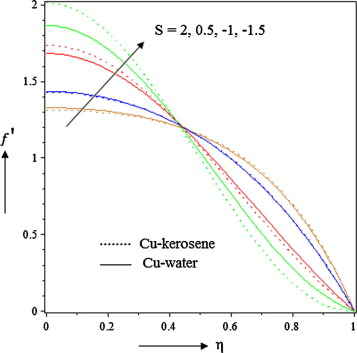Figure 2. Effects of squeezing parameter S on velocity.

Table 5. Effects of S   on ${\textstyle {Cf}_{r}}$ and ${\textstyle {Nu}_{r}}$.
S ${\textstyle {Cf}_{r}}$ ${\textstyle {Nu}_{r}}$
Cu-water Cu-kerosene Cu-water Cu-kerosene
−1.0 −1.938344 −1.819084 1.225071 1.232899
0.5 −3.096871 −2.983827 1.137759 1.138204
1.0 −3.390695 −3.275727 1.119464 1.111067

Fig. 3 shows that the nanofluid temperature decreases as S   increases. Since ${\textstyle S={\frac {\alpha H^{2}}{2{\nu }_{f}}}}$ hence increase in the squeezing parameter S represents the decrease in kinematic viscosity as well as the speed at which the plates move apart. Consequently the friction between the boundary surface of the plates and nanoparticles reduces and so the temperature of the nanofluid. This result is found to be identical with Mustafa et al. . Table 5 shows that Nusselt number ${\textstyle {Nu}_{r}}$ decreases with increasing S for both Cu-water and Cu-kerosene.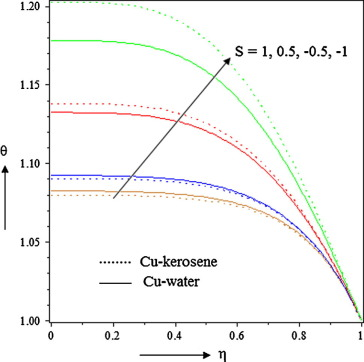Figure 3. Effects of squeezing parameter S on temperature.

### 4.2. Effect of φ on velocity and temperature profiles

Figure 4(a) and Figure 4(b) depict the effect nanoparticle volume fraction φ on nanofluid velocity for S > 0 and S < 0. It has been found that for ${\textstyle 0\leqslant \eta \leqslant 0.42}$ (not accurately determined) velocity increases as φ   increases but the effect is opposite for ${\textstyle \eta >0.42}$ (not accurately determined). The nature of the effect is same for both for S > 0 and S < 0. But when two plates are moving apart i.e., S > 0 then the velocity of Cu-kerosene is less as compared to Cu-water within the region ${\textstyle 0\leqslant \eta \leqslant 0.42{\mbox{.}}}$ However the effect is opposite and prominent when S < 0 i.e., so-called squeezing flow. From Table 6 it is seen that the influence of φ   increases the reduced skin friction coefficient ${\textstyle {Cf}_{r}}$ for both Cu-water and Cu-kerosene.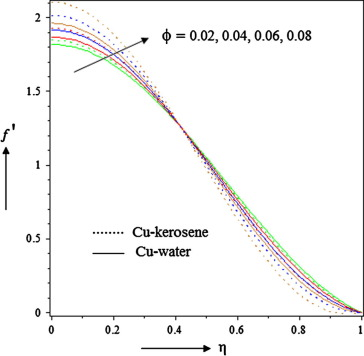Figure 4(a). Effect of nanoparticle volume fraction on velocity when S < 0.Figure 4(b). Effect of nanoparticle volume fraction on velocity when S > 0.

Table 6. Effects of ${\textstyle \phi }$, M   on ${\textstyle {Cf}_{r}}$.
${\textstyle \phi }$ M ${\textstyle {Cf}_{r}}$
Cu-water Cu-kerosene
S = 0.5 S = −0.5 S = 0.5 S = −0.5
0.02 0.5 −3.096871 −2.388320 −2.983826 −2.274793
0.04 −2.889905 −2.180419 −2.711130 −2.000632
0.06 −2.736134 −2.025792 −2.515986 −1.804083
0.02 1.5 −3.402609 −2.773329 −3.275986 −2.646386
3 −4.277192 −3.811202 −4.112513 −3.646416
4.5 −5.414585 −5.074220 −5.201616 −4.861222

We have represented the temperature profiles against η for various values of nanoparticle volume fraction φ in Figure 5(a) and Figure 5(b) for both S < 0 and S > 0 respectively. Fig. 5(a) shows that the fluid temperature possesses high value because of increasing values of φ for S < 0 i.e., the squeezing flow. The reason behind the phenomena is that when two plates move to each other and nanoparticle volume increases then there are more collision between nanoparticles and particles with the boundary surface of the plate and consequently the resulting friction gives rise to increase the temperature within the fluid near the boundary region. It should be noted that fluid temperature of Cu-kerosene is significantly increased as compared to Cu-water. Higher values of Prandtl number of kerosene are responsible for this phenomenon. But the result is totally opposite for S > 0 i.e., when two plates move apart. On the other hand Nusselt number increases with φ for both nanofluids as shown in Table 7.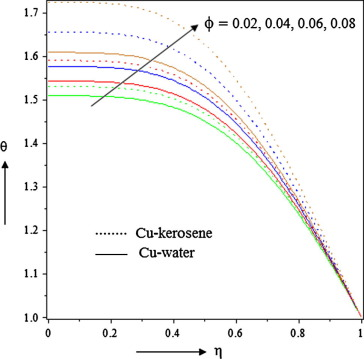Figure 5(a). Effect of nanoparticle volume fraction on temperature when S < 0.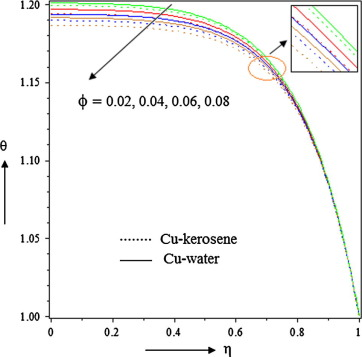Figure 5(b). Effect of nanoparticle volume fraction on temperature when S > 0.

Table 7. Effects of ${\textstyle \phi }$, M  , Ec on ${\textstyle {Nu}_{r}}$.
${\textstyle \phi }$ M Ec ${\textstyle {Nu}_{r}}$
Cu-water Cu-kerosene
S = 0.5 S = −0.5 S = 0.5 S = −0.5
0.02 0.5 0.5 1.137759 1.160594 1.138203 1.162025
0.04 1.199843 1.223263 1.200806 1.226410
0.06 1.266466 1.290603 1.267997 1.295712
0.02 1.5 1.157179 1.149260 1.157937 1.149597
3.0 1.251637 1.206060 1.252779 1.205117
4.5 1.426942 1.376159 1.428062 1.375016
0.02 0.5 0.5 2.275519 2.321188 2.276407 2.324051
1.5 3.413278 3.481782 3.414611 3.486075
2.5 4.551038 4.642375 4.552815 4.648101

### 4.3. Effect of M on velocity and temperature profiles

The impact of Hartmann number M on velocity distribution has been portrayed in Figure 6(a) and Figure 6(b) for both S > 0 and S < 0. It reveals that as M   increases the velocity decreases for ${\textstyle 0\leqslant \eta \leqslant 0.42}$ (not accurately determined) and it is true because for electrically conducting fluid in the presence of magnetic field there will always be Lorentz force which slows the motion of fluid in the boundary layer region. But for ${\textstyle \eta >0.42}$ (not accurately determined) the reverse effect is seen because of the same mass flow reason as discussed in the previous Section 4.1. Moreover when S < 0 two plates are very close to each other, then the situation together with retarding Lorentz force creates adverse pressure gradient. Whenever such forces act over a long time then there might be a point of separation and back flow occurs. For S > 0 the reason is slightly different. When two plates move apart then a vacant space occurs and fluid in that region goes with high velocity so that mass flow conservation will not be violated. That’s why for ${\textstyle \eta >0.42}$ we suddenly find an accelerated flow. For ${\textstyle 0\leqslant \eta \leqslant 0.42}$ velocity of Cu-water is less as compared to Cu-kerosene for S < 0 whereas the opposite effect occurs for S > 0. It should be noted from Table 6 that reduced skin friction coefficient ${\textstyle {Cf}_{r}}$ decreases as M goes high.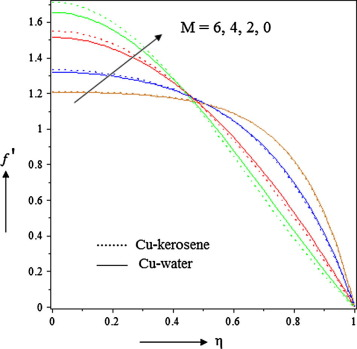Figure 6(a). Effects of Hartmann number M on velocity when S < 0.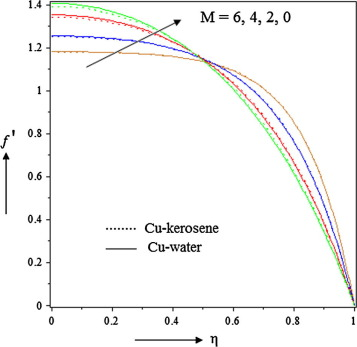Figure 6(b). Effects of Hartmann number M on velocity when S > 0.

Figure 7(a) and Figure 7(b) exhibits that the temperature of the nanofluid decreases as Hartmann number increases for both S < 0 and S > 0. For S < 0 the effect is more prominent and temperature of Cu-kerosene is higher than Cu-water nanofluid. The effect is opposite for S > 0. It is worth mentioning that for S > 0 we see a crossover of temperature profile at η = 0.82 (not accurately determined). But after that region the effect is negligible. From Table 7 we see that Nusselt number increases as M increases.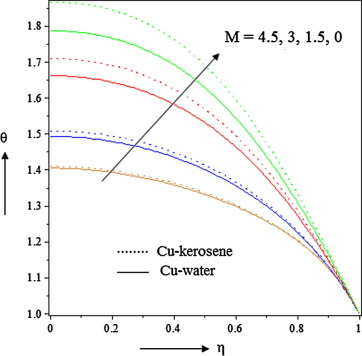Figure 7(a). Effects of Hartmann number M on temperature when S < 0.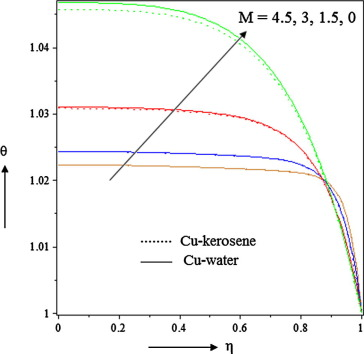Figure 7(b). Effects of Hartmann number M on temperature when S > 0.

### 4.4. Effect of Eckert number on temperature profiles

The influence of Eckert number Ec on nanofluid temperature profile has been portrayed in Figure 8(a) and Figure 8(b) for both S > 0 and S < 0. It reveals that the temperature significantly increases with the increasing value of Ec by means of frictional heating. Also the Nusselt number increases as Ec increases for both S > 0 and S < 0 as shown in Table 7. It should be noted that Cu-kerosene possess higher temperature than Cu-water for S < 0. But the reverse effect is seen for S > 0.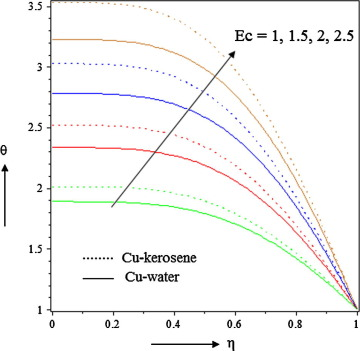Figure 8(a). Effects of Eckert number Ec on temperature when S < 0.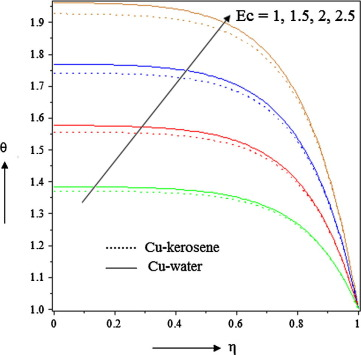Figure 8(b). Effects of Eckert number Ec on temperature when S > 0.

## 5. Conclusion

In the present paper the influence of magnetic field on squeezing flow of Cu-water and Cu-kerosene nanofluids has been discussed. The model is transformed and rendered into dimensionless form and then solved analytically using differential transformation method (DTM) as well as numerically using RK-4 together with comparison to show the efficiency of DTM. The numerical discussion has been performed through graphs and tables to illustrate the details of the flow characteristics. Based on the whole discussion the main conclusions of our investigation are as follows.

It is observed that fluid velocity decreases with the influence of squeezing parameter and Hartmann number within the region ${\textstyle 0\leqslant \eta \leqslant 0.42{\mbox{.}}}$ But fluid velocity increases within the same region due to nanoparticle volume fraction φ   whereas opposite effect is seen in both cases for ${\textstyle \eta >0.42{\mbox{.}}}$.

• The enhanced Hartmann number and Eckert number allow to increase the Nusselt number for both Cu-water and Cu-kerosene nanofluids. But it is a decreasing function of squeeze number.
• When S > 0 i.e., when two plates are moving apart, then the velocity of Cu-kerosene is less as compared to Cu-water. However, opposite effect is observed for S < 0 i.e., when two plates are moving to each other.
• Considering the situation S > 0 leads to decrease the temperature of the fluid when nanoparticle volume fraction and Hartmann increase. But the impact of Eckert number is opposite here. It is seen that the temperature of Cu-kerosene is less always as compared to Cu-water in this situation. On the other hand the effect is totally reverse for S < 0.
• The results indicate a direct relationship between fluid velocity and skin friction coefficient but enhanced squeeze number decreases the skin friction coefficient.

## Acknowledgment

The authors wish to express their sincere thanks to the honorable reviewers for the valuable comments and suggestions to improve the quality of the paper.

### Document informationPublished on 12/04/17

Licence: Other

### Document Score0

Views 105
Recommendations 0